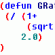# Draw Rectangle Dimension## Recommended PostsHello! I was wondering how I would go about writing a function that would calculate the length of a diagonal of a rectangle using SQRT function? I've been trying but so far nothing!

Thanks!

##### Share on other sitesCan you post the code you wrote so far?

Presuming that you know the length, respectively width of the rectangle, you should use Pythagoras formula:

`(setq sizeDiagonal (sqrt (+ (expt sizeLength 2.0) (expt sizeWidth 2.0))))`

##### Share on other sitesThanks! I had a few simple lines, but I erased those as yours was basically what I needed. Would it be possible to write a function that would find the length & width itself? This one is out of curiosity.

##### Share on other sitesThanks! I had a few simple lines, but I erased those as yours was basically what I needed. Would it be possible to write a function that would find the length & width itself? This one is out of curiosity.

Try this:

```(defun LM:MAssoc ( key lst / item )
(if (setq item (assoc key lst))
(cons (cdr item) (LM:MAssoc key (cdr (member item lst))))
)
)
(defun c:getdist (/ s pt1 pt2 pt3 pt4 x y ent lst)
(setq s (ssget "_+.:E:S" '((0 . "LWPOLYLINE"))))
(if (= s nil)
(progn
(setq ent  (entget(ssname s 0)))
(setq lst (LM:MAssoc 10 ent))
(princ)
(setq pt1 (nth 0 lst))
(setq pt2 (nth 1 lst))
(setq pt3 (nth 2 lst))
(setq pt4 (nth 3 lst))
(setq x(distance pt1 pt2))
(setq y(distance pt1 pt4))
(princ (strcat "\nHeight: " (rtos y) "\nWidth: " (rtos x)))
(princ)
)
)
)
```

Yes, thanks to Lee Mac haha

Edit: But there is some problem with this code, if you rotate your rectangle by 90º the height will become width and vice versa.

Nothing that a if can't solve hehe

Edited by fabriciorby
##### Share on other sitesHere is another method:

```(defun c:test ( / lst sel )
(if (setq sel (ssget "_+.:E:S" '((0 . "LWPOLYLINE"))))
(progn
(setq lst
(mapcar 'cdr
(vl-remove-if-not '(lambda ( x ) (= 10 (car x)))
(entget (ssname sel 0))
)
)
)
(apply 'mapcar
(cons '-
(mapcar
'(lambda ( x ) (apply 'mapcar (cons x lst)))
'(max min)
)
)
)
)
)
)```

## Join the conversation

You can post now and register later. If you have an account, sign in now to post with your account.
Note: Your post will require moderator approval before it will be visible.Unfortunately, your content contains terms that we do not allow. Please edit your content to remove the highlighted words below.

×   Pasted as rich text.   Restore formatting

Only 75 emoji are allowed.